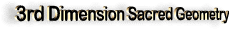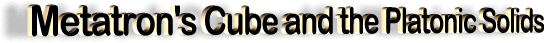by IDTimeTraveller

from 3rdDimensionOnLine Website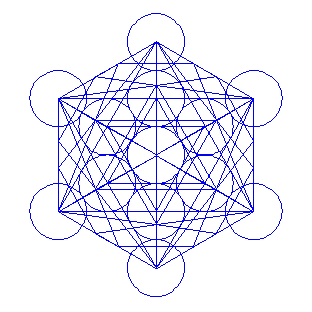Metatron's Cube

The image you see above is the correct version of "Metatron’s Cube", or the fruit of life with the 5 platonic solids. I made it with a 3D program and what you see are the actual edges of the platonic solids.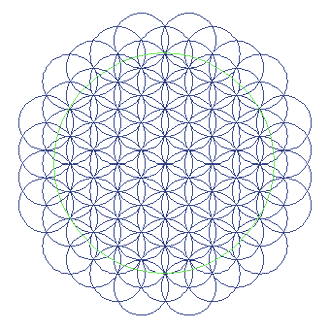The dodecahedron and icosahedron actually have their "inner" corner points touching the star tetrahedron that fits in a circle that is = 1/Ø x the circle connecting the corners of the outer cube.

Where Ø ("phi") = 1.618 033 988 749 594 ...

The way to figure out drawing this is by beginning with the normal flower of life "grid" (of 5 non-intersecting inner circles, or in this case: 7 intersecting full circles - left image):

Then draw a circle that is equal to 1/Ø x the outer circle.

For this, put the point of a compass on the middle of the vertical radius (in the centre of the second "flower" from the top along the vertical axis) and draw a circle from where the horizontal axis (through the middle of the circle) cuts the outer circle (see below);
The circle you can draw when you put one point of the compass in the centre of the grid, and draw a circle from where the other circle cuts the vertical axis (through the middle of the circle), is a circle Ø times smaller than the outer circle: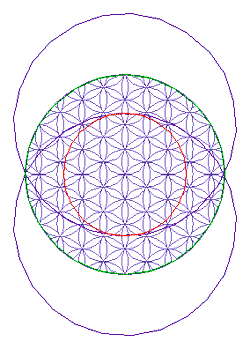After this, draw the appropriate startetrahedron(s) and you have found all the points necessary to draw the other platonic solids.

Here are all the platonic solids the way they fit in Metatron’s cube: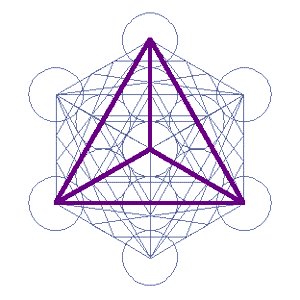Tetrahedron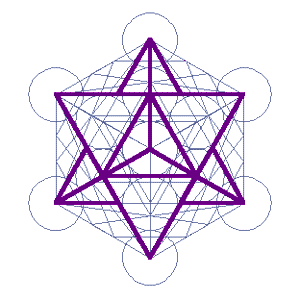Star tetrahedron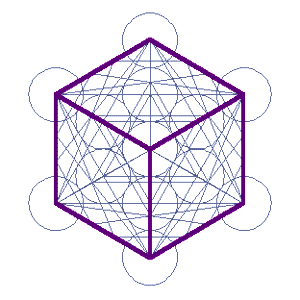Cube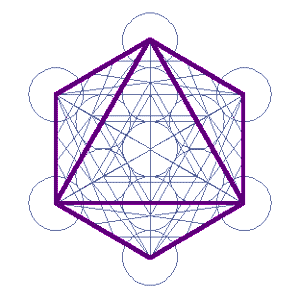Octahedron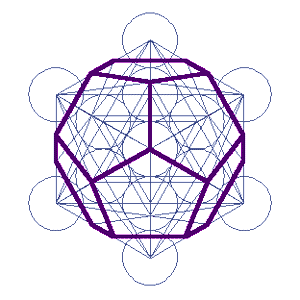Dodecahedron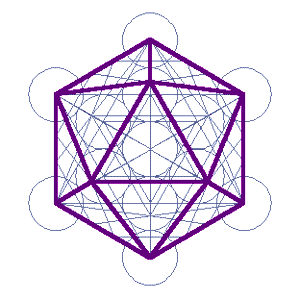Icosahedron

All platonic solids

Not all solids can be (fully) seen, as some lines are hidden behind others.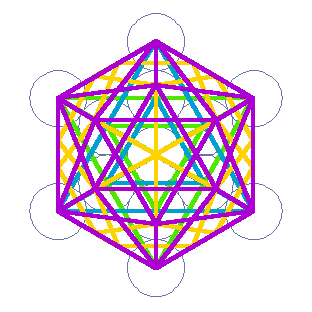All solids as in metatron’s cube, seen from side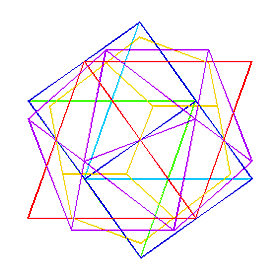Here are all the solids of Metatron’s cube. This is what you see in Metatron’s cube from the top, but here it is seen from the (right) side. Classic representation of dodecahdron in tetrahedron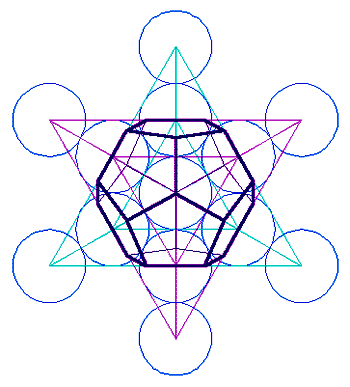This is a correct version of a dodecahdron in a startetrahedron. It’s a classic representation that is commonly done in a way that is incorrect.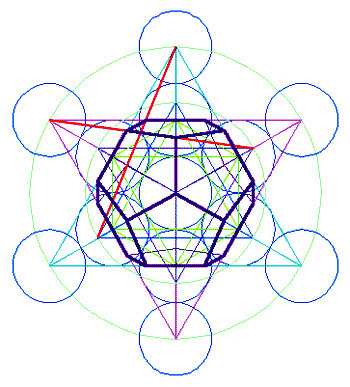The corners of the dodecahedron are found by drawing lines from the corners of the big startetrahedron to those of a startetrahedron that is Ø times smaller. .

from Sangraal Website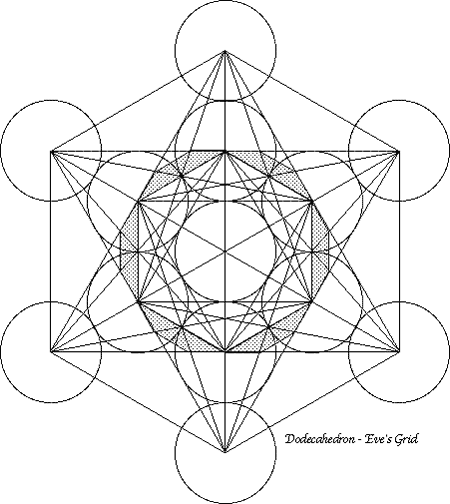Drawing by Drunvalo Melchizedek

Perhaps you can see the rectangle with the triad on top and bottom. It is part of the cube nested within a cube. Metatron’s Cube is the incubator of 3D. It represents a box within a box or a cube nested within a cube.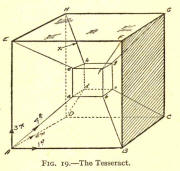The Tesseract Drawing comes from a book published in 1928 titled "Theosophy and the Fourth Dimension" by Alexander Horne.

The Tesseract represents a process in which one might peer into the fourth dimension. The drawings of the Dodecahedron - Eve’s Grid and the Tesseract are basically the same geometry except that the Tesseract is tilted so you might better define the six sections.

Outlined in red is one of the six sections. The idea is to go into the outlined area and see it as a cube. Once you have visualized being inside the cube, it represents the cube of 4D. Click on the Tesseract to get a larger image for visualization.

It often takes a student several tries to actually get inside the 4D cube. The larger image works much better than the small one. If you have difficulty after a couple of days, perhaps stop for a few days and try again. It took me several attempts to see it the first time.

Seeing the cube within a cube dimensionally leads one to consider the nature of the Holographic model. It has the nature of being exactly the same structure if you were to tear off a piece from the edge. The integrity of the geometry would be maintained infinitely. The image below could be that of the corner you just ripped off the edge.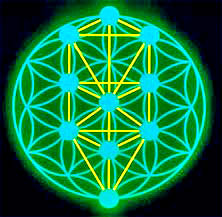Above, we see that the Tree of Life is contained in Metatron’s Cube/Grid. The view above comes from the Seed of Life, therefore metaphorically, the Tree is contained within the seed, and is a fractal property from the beginning.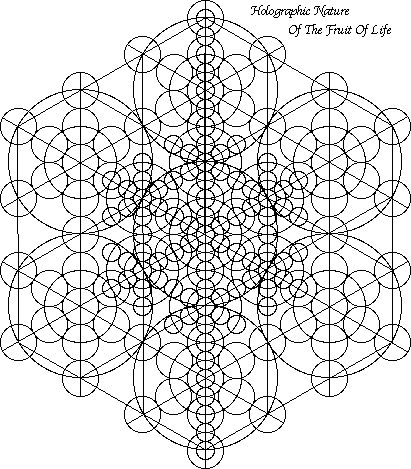Drawing by Drunvalo Melchizedek

When considering the Flower of life Geometries, keep in mind that curved lines represent female characteristics and straight lines represent male characteristics. The drawing depicts the holographic nature of the male and female character of the grid work and a deeper, more "within" nature, of Metatron’s Cube.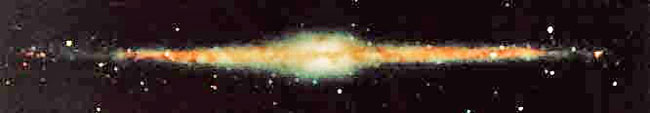Merkaba Field